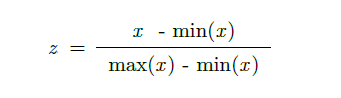# What is min-max normalization? Where do we use it?

Min-max normalization

Min-Max Normalization is a type of normalization.
This method rescales the range of the data to [0,1].
In most cases, standardization is used feature-wise as well.

Min-max normalisation is often known as feature scaling where the values of a numeric range of a feature of data, i.e. a property, are reduced to a scale between 0 and 1. Therefore, in order to calculate z, i.e. the normalised value of a member of the set of observed values of x, we must employ the following formula:Where min and max are the minimum and maximum values in x given its range.